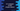# C++ program to find simple interest## C++ program to find simple interest :

In this C++ example program, we will learn how to find the simple interest. The user will enter the inputs and it will calculate the simple interest and print it out to the user.

To calculate the simple interest, three values are required: principal amount, time, and rate of interest. With these values, we can calculate the simple interest by using the following formula :

``(principal amount * time * rate of interest)/100``

Our program will take these values as input from the user.

### C++ program :

``````#include <iostream>
using namespace std;

//1
int main()
{
//2
float N, P, R;
float SI;

//3
cout << "Enter the principal amount : " << endl; cin >> P;

//4
cout << "Enter the time in years : " << endl; cin >> N;

//5
cout << "Enter the rate of interest : " << endl; cin >> R;

//6
SI = (N * P * R) / 100;

//7
cout << "Simple Interest : " << SI << endl;
return 0;
}``````

### Explanation :

The commented numbers in the above program denote the step numbers below :

1. main() is the starting point of a C++ program. It executes at the start.
2. N, P and R are floating point variables we have created to hold the interest time, principal amount and rate of interest respectively.
3. Ask the user to enter the principal amount. Read and store it in P.
4. Ask the user to enter the interest time in years. Read and store it in N.
5. Ask the user to enter the rate of interest. Read and store it in R.
6. Calculate the simple interest and store it in the SI variable.
7. Print out the simple interest or SI to the user.

### Sample Output :

``````Enter the principal amount :
1200
Enter the time in years :
12
Enter the rate of interest :
10
Simple Interest : 1440``````